Ch 1. Basics Multimedia Engineering Statics Problem Solving Units
 Chapter 1. Basics 2. Vectors 3. Forces 4. Moments 5. Rigid Bodies 6. Structures 7. Centroids/Inertia 8. Internal Loads 9. Friction 10. Work & Energy Appendix Basic Math Units Sections Search eBooks Dynamics Fluids Math Mechanics Statics Thermodynamics Author(s): Kurt Gramoll ©Kurt GramollSTATICS - CASE STUDY SOLUTION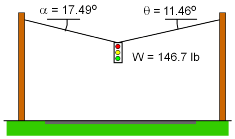Using the basic force-equilibrium equations in the x and y directions, the forces FA and FB can be determined:      ΣFx = 0      ΣFy = 0 Sum the forces in both directions gives:      -FA cosα + FB cosθ = 0      FA sinα + FB sinθ - W = 0 Four Significant Digits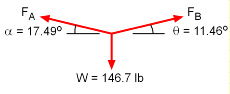Substitute numerical values up to four significant digits into the previous two equations.      -FA cos17.49 + FB cos11.46 = 0       FA sin17.49 + FB sin11.46 -146.7 = 0 Solve the two equations for the unknown values of FA and FB: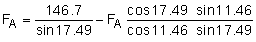FA   = 488.1 - 0.6434 FA      FA   = 297.0 lb      FB   = 289.0 lb Two Significant Digits Round the given numerical values to two significant digits and substitute into previous equations.      -FA cos17 + FB cos11 = 0      FA sin17 + FB sin11 - 150 = 0 Solve the two equations for the unknown values of FA and FB: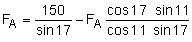The numerical values in each step are also rounded to two significant digits: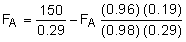FA   = 520 - 0.64 FA      FA   = 320 lb      FB   = 320 lb Notice the value of FA is 23 lb, or 7.7% higher, than when four significant digits were used. The percentage of error will vary from problem to problem, but when only two significant digits are used, the answer will generally be off by more than 1 percent. Therefore, rounding to only two significant digits should be avoided.

Practice Homework and Test problems now available in the 'Eng Statics' mobile app
Includes over 500 free problems with complete detailed solutions.
Available at the Google Play Store and Apple App Store.